# 9- COORDINATE COMPOUNDS

Here we are going through the ncert solutions for class 12 chemistry chapter 9- coordinate compound so before going through the ncert solutions make sure to go through the textbook that helps you to understand the solutions more easily

# ​​ ​​ ​​ ​​ ​​ ​​ ​​ ​​ ​​ ​​ ​​ ​​ ​​ ​​ ​​ ​​ ​​ ​​ ​​ ​​ ​​ ​​ ​​ ​​ ​​ ​​ ​​ ​​ ​​ ​​ ​​ ​​ ​​ ​​ ​​ ​​ ​​ ​​ ​​ ​​ ​​ ​​ ​​ ​​ ​​ ​​ ​​​​ INTEXT ANSWERS

#### Question 9.1:

Write the formulas for the following coordination compounds:

(i) Tetraamminediaquacobalt(III) chloride

(ii) Potassium tetracyanonickelate(II)

(iii) Tris(ethane−1,2−diamine) chromium(III) chloride

(iv) Amminebromidochloridonitrito-N-platinate(II)

(v) Dichloridobis(ethane−1,2−diamine)platinum(IV) nitrate

(vi) Iron(III) hexacyanoferrate(II)

(i)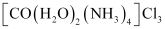(ii)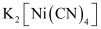(iii)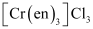(vi)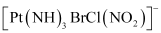(v)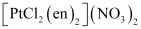(vi)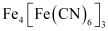#### Question 9.2:

Write the IUPAC names of the following coordination compounds:

(i) [Co(NH3)6]Cl3

(ii) [Co(NH3)5Cl]Cl2

(iii) K3[Fe(CN)6]

(iv) K3[Fe(C2O4)3]

(v) K2[PdCl4]

(vi) [Pt(NH3)2Cl(NH2CH3)]Cl

(i) Hexaamminecobalt(III) chloride

(ii) Pentaamminechloridocobalt(III) chloride

(iii) Potassium hexacyanoferrate(III)

(iv) Potassium trioxalatoferrate(III)

(vi) Diamminechlorido(methylamine)platinum(II) chloride

#### Question 9.3:

Indicate the types of isomerism exhibited by the following complexes and

draw the structures for these isomers:

• K[Cr(H2O)2(C2O4)2

• [Co(en)3]Cl3

• [Co(NH3)5(NO2)](NO3)2

• [Pt(NH3)(H2O)Cl2]

• Both geometrical (cis-, trans-) isomers for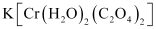can exist. Also, optical isomers for cis-isomer exist.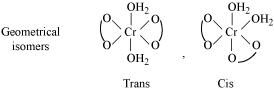Trans-isomer is optically inactive. On the other hand, cis-isomer is optically active.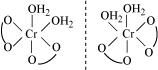(ii) Two optical isomers for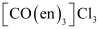exist.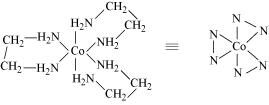Two optical isomers are possible for this structure.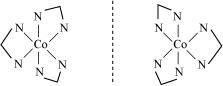(iii)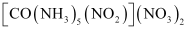A pair of optical isomers: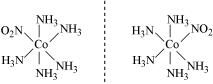It can also show linkage isomerism.and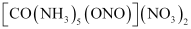It can also show ionization isomerism.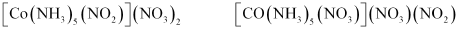(iv) Geometrical (cis-trans-) isomers of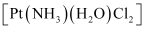can exist.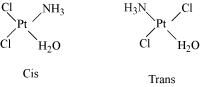#### Question 9.4:

Give evidence that [Co(NH3)5Cl]SO4 and [Co(NH3)5SO4]Cl are ionization isomers.

When ionization isomers are dissolved in water, they ionize to give different ions. These ions then react differently with different reagents to give different products.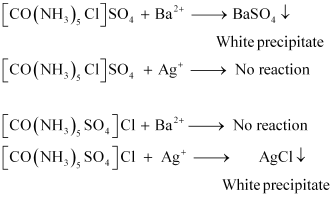Question 9.5:

Explain on the basis of valence bond theory that [Ni(CN)4]2− ion with square

planar structure is diamagnetic and the [NiCl4]2− ion with tetrahedral geometry is paramagnetic.

Ni is in the +2 oxidation state i.e., in d8 configuration.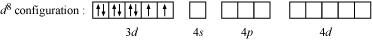There are 4 CN ions. Thus, it can either have a tetrahedral geometry or square planar geometry. Since CN ion is a strong field ligand, it causes the pairing of unpaired 3d electrons.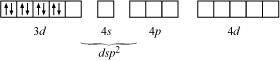It now undergoes dsp2 hybridization. Since all electrons are paired, it is diamagnetic.

In case of [NiCl4]2−, Cl ion is a weak field ligand. Therefore, it does not lead to the pairing of unpaired 3d electrons. Therefore, it undergoes sp3 hybridization.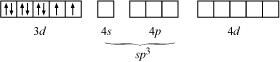Since there are 2 unpaired electrons in this case, it is paramagnetic in nature.

#### Question 9.6:

[NiCl4]2− is paramagnetic while [Ni(CO)4] is diamagnetic though both are tetrahedral. Why?

Though both [NiCl4]2− and [Ni(CO)4] are tetrahedral, their magnetic characters are different. This is due to a difference in the nature of ligands. Cl is a weak field ligand and it does not cause the pairing of unpaired 3d electrons. Hence, [NiCl4]2− is paramagnetic.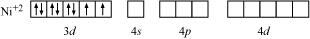In Ni(CO)4, Ni is in the zero oxidation state i.e., it has a configuration of 3d8 4s2.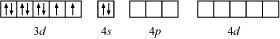But CO is a strong field ligand. Therefore, it causes the pairing of unpaired 3d electrons. Also, it causes the 4s electrons to shift to the 3d orbital, thereby giving rise to sp3 hybridization. Since no unpaired electrons are present in this case, [Ni(CO)4] is diamagnetic.

#### Question 9.7:

[Fe(H2O)6]3+ is strongly paramagnetic whereas [Fe(CN)6]3− is weakly paramagnetic. Explain.

In both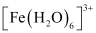and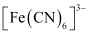, Fe exists in the +3 oxidation state i.e., in d5 configuration.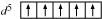Since CN is a strong field ligand, it causes the pairing of unpaired electrons. Therefore, there is only one unpaired electron left in the d-orbital.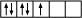Therefore,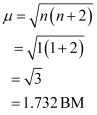On the other hand, H2O is a weak field ligand. Therefore, it cannot cause the pairing of electrons. This means that the number of unpaired electrons is 5.

Therefore,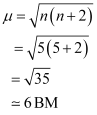Thus, it is evident thatis strongly paramagnetic, whileis weakly paramagnetic.

#### Question 9.8:

Explain [Co(NH3)6]3+ is an inner orbital complex whereas [Ni(NH3)6]2+ is an outer orbital complex.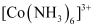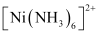Oxidation state of cobalt = +3 Oxidation state of Ni = +2 Electronic configuration of cobalt = d6 Electronic configuration of nickel = d8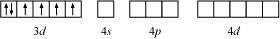NH3 being a strong field ligand causes the pairing. Therefore, Cobalt can undergo d2sp3 hybridization.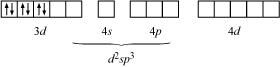Hence, it is an inner orbital complex.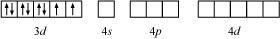If NH3 causes the pairing, then only one 3d orbital is empty. Thus, it cannot undergo d2sp3 hybridization. Therefore, it undergoes sp3d2 hybridization.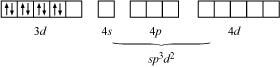Hence, it forms an outer orbital complex.

#### Question 9.9:

Predict the number of unpaired electrons in the square planar [Pt(CN)4]2− ion.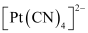In this complex, Pt is in the +2 state. It forms a square planar structure. This means that it undergoes dsp2 hybridization. Now, the electronic configuration of Pd(+2) is 5d8.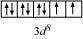CN being a strong field ligand causes the pairing of unpaired electrons. Hence, there are no unpaired electrons in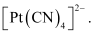#### Question 9.10:

The hexaquo manganese(II) ion contains five unpaired electrons, while the hexacyanoion contains only one unpaired electron. Explain using Crystal Field Theory.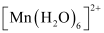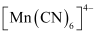Mn is in the +2 oxidation state. Mn is in the +2 oxidation state. The electronic configuration is d5. The electronic configuration is d5. The crystal field is octahedral. Water is a weak field ligand. Therefore, the arrangement of the electrons inis t2g3eg2. The crystal field is octahedral. Cyanide is a strong field ligand. Therefore, the arrangement of the electrons inisT2g5eg0.

Hence, hexaaquo manganese (II) ion has five unpaired electrons, while hexacyano ion has only one unpaired electron.

Question 9.11:

Calculate the overall complex dissociation equilibrium constant for the Cu(NH3)42+ ion, given that β4 for this complex is 2.1 × 1013.

β= 2.1 × 1013

The overall complex dissociation equilibrium constant is the reciprocal of the overall stability constant, β4.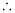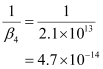#### Question 9.1:

Explain the bonding in coordination compounds in terms of Werner’s postulates.

Werner’s postulates explain the bonding in coordination compounds as follows:

(i) A metal exhibits two types of valencies namely, primary and secondary valencies. Primary valencies are satisfied by negative ions while secondary valencies are satisfied by both negative and neutral ions.

(In modern terminology, the primary valency corresponds to the oxidation number of the metal ion, whereas the secondary valency refers to the coordination number of the metal ion.

(ii) A metal ion has a definite number of secondary valencies around the central atom. Also, these valencies project in a specific direction in the space assigned to the definite geometry of the coordination compound.

(iii) Primary valencies are usually ionizable, while secondary valencies are non-ionizable.

#### Question 9.2:

FeSO4 solution mixed with (NH4)2SO4 solution in 1:1 molar ratio gives the test of Fe2+ ion but CuSOsolution mixed with aqueous ammonia in 1:4 molar ratio does not give the test of Cu2+ ion. Explain why?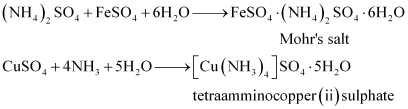Both the compounds i.e.,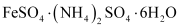and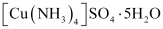fall under the category of addition compounds with only one major difference i.e., the former is an example of a double salt, while the latter is a coordination compound.

A double salt is an addition compound that is stable in the solid state but that which breaks up into its constituent ions in the dissolved state. These compounds exhibit individual properties of their constituents. For e.g.breaks into Fe2+, NH4+, and SO42− ions. Hence, it gives a positive test for Fe2+ ions.

A coordination compound is an addition compound which retains its identity in the solid as well as in the dissolved state. However, the individual properties of the constituents are lost. This happens becausedoes not show the test for Cu2+. The ions present in the solution ofare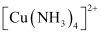and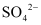.

#### Question 9.3:

Explain with two examples each of the following: coordination entity, ligand, coordination number, coordination polyhedron, homoleptic and heteroleptic.

(i) Coordination entity:

A coordination entity is an electrically charged radical or species carrying a positive or negative charge. In a coordination entity, the central atom or ion is surrounded by a suitable number of neutral molecules or negative ions ( called ligands). For example: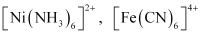= cationic complex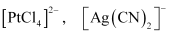= anionic complex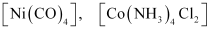= neutral complex

(ii) Ligands

The neutral molecules or negatively charged ions that surround the metal atom in a coordination entity or a coordinal complex are known as ligands. For example,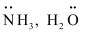, ClOH. Ligands are usually polar in nature and possess at least one unshared pair of valence electrons.

(iii) Coordination number:

The total number of ligands (either neutral molecules or negative ions) that get attached to the central metal atom in the coordination sphere is called the coordination number of the central metal atom. It is also referred to as its ligancy.

For example:

(a) In the complex, K2[PtCl6], there as six chloride ions attached to Pt in the coordinate sphere. Therefore, the coordination number of Pt is 6.

(b) Similarly, in the complex [Ni(NH3)4]Cl2, the coordination number of the central atom (Ni) is 4.

(vi) Coordination polyhedron:

Coordination polyhedrons about the central atom can be defined as the spatial arrangement of the ligands that are directly attached to the central metal ion in the coordination sphere. For example:

(a)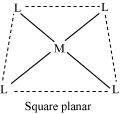(b) Tetrahedral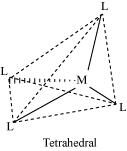(v) Homoleptic complexes:

These are those complexes in which the metal ion is bound to only one kind of a donor group. For eg: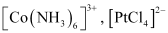etc.

(vi) Heteroleptic complexes:

Heteroleptic complexes are those complexes where the central metal ion is bound to more than one type of a donor group.

For e.g.: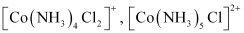#### Question 9.4:

What is meant by unidentate, didentate and ambidentate ligands? Give two examples for each.

A ligand may contain one or more unshared pairs of electrons which are called the donor sites of ligands. Now, depending on the number of these donor sites, ligands can be classified as follows:

(a) Unidentate ligands: Ligands with only one donor sites are called unidentate ligands. For e.g.,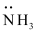, Cl etc.

(b) Didentate ligands: Ligands that have two donor sites are called didentate ligands. For e.g.,

(a) Ethane-1,2-diamine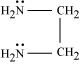(b) Oxalate ion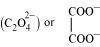(c) Ambidentate ligands:

Ligands that can attach themselves to the central metal atom through two different atoms are called ambidentate ligands. For example:

(a)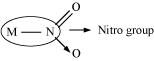(The donor atom is N)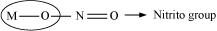(The donor atom is oxygen)

(b)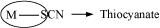(The donor atom is S)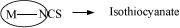(The donor atom is N)

#### Question 9.5:

Specify the oxidation numbers of the metals in the following coordination entities:

(i) [Co(H2O)(CN)(en)2]2+

(ii) [CoBr2(en)2]+

(iii) [PtCl4]2−

(iv) K3[Fe(CN)6]

(v) [Cr(NH3)3Cl3]

(i)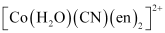Let the oxidation number of Co be x.

The charge on the complex is +2.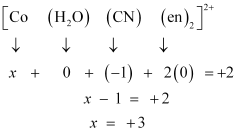(ii)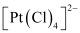Let the oxidation number of Pt be x.

The charge on the complex is −2.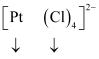x + 4(−1) = −2

x = + 2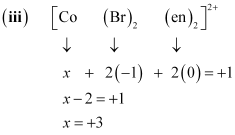(iv)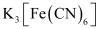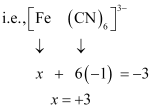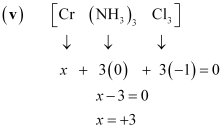#### Question 9.6:

Using IUPAC norms write the formulas for the following:

(i) Tetrahydroxozincate(II)

(iii) Diamminedichloridoplatinum(II)

(iv) Potassium tetracyanonickelate(II)

(v) Pentaamminenitrito-O-cobalt(III)

(vi) Hexaamminecobalt(III) sulphate

(vii) Potassium tri(oxalato)chromate(III)

(viii) Hexaammineplatinum(IV)

(ix) Tetrabromidocuprate(II)

(x) Pentaamminenitrito-N-cobalt(III)

(i) [Zn(OH)4]2−

(ii) K2[PdCl4]

(iii) [Pt(NH3)2Cl2]

(iv) K2[Ni(CN)4]

(v) [Co(ONO) (NH3)5]2+

(vi) [Co(NH3)6]2 (SO4)3

(vii) K3[Cr(C2O4)3]

(viii) [Pt(NH3)6]4+

(ix) [Cu(Br)4]2−

(x) [Co[NO2)(NH3)5]2+

#### Question 9.7:

Using IUPAC norms write the systematic names of the following:

(i) [Co(NH3)6]Cl3

(ii) [Pt(NH3)2Cl(NH2CH3)]Cl

(iii) [Ti(H2O)6]3+

(iv) [Co(NH3)4Cl(NO2)]Cl

(v) [Mn(H2O)6]2+

(vi) [NiCl4]2−

(vii) [Ni(NH3)6]Cl2

(viii) [Co(en)3]3+

(ix) [Ni(CO)4]

(i) Hexaamminecobalt(III) chloride

(ii) Diamminechlorido(methylamine) platinum(II) chloride

(iii) Hexaquatitanium(III) ion

(iv) Tetraamminichloridonitrito-N-Cobalt(III) chloride

(v) Hexaquamanganese(II) ion

(vi) Tetrachloridonickelate(II) ion

(vii) Hexaamminenickel(II) chloride

(viii) Tris(ethane-1, 2-diammine) cobalt(III) ion

(ix) Tetracarbonylnickel(0)

#### Question 9.8:

List various types of isomerism possible for coordination compounds, giving an example of each.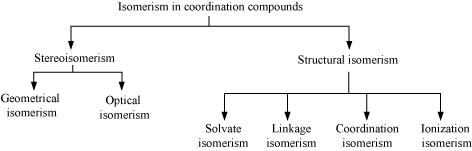(a) Geometric isomerism:

This type of isomerism is common in heteroleptic complexes. It arises due to the different possible geometric arrangements of the ligands. For example: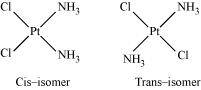(b) Optical isomerism:

This type of isomerism arises in chiral molecules. Isomers are mirror images of each other and are non-superimposable.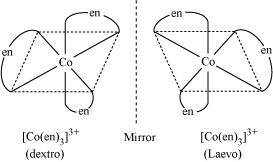(c) Linkage isomerism: This type of isomerism is found in complexes that contain ambidentate ligands. For example:

[Co(NH3)5 (NO2)]Cl2 and [Co(NH3)5 (ONO)Cl2

Yellow form Red form

(d) Coordination isomerism:

This type of isomerism arises when the ligands are interchanged between cationic and anionic entities of differnet metal ions present in the complex.

[Co(NH3)6] [Cr(CN)6] and [Cr(NH3)6] [Co(CN)6]

(e) Ionization isomerism:

This type of isomerism arises when a counter ion replaces a ligand within the coordination sphere. Thus, complexes that have the same composition, but furnish different ions when dissolved in water are called ionization isomers. For e.g., Co(NH3)5SO4)Br and Co(NH3)5Br]SO4.

(f) Solvate isomerism:

Solvate isomers differ by whether or not the solvent molecule is directly bonded to the metal ion or merely present as a free solvent molecule in the crystal lattice.

[Cr[H2O)6]Cl3 [Cr(H2O)5Cl]Cl2H2O [Cr(H2O)5Cl2]Cl2H2O

Violet Blue-green Dark green

#### Question 9.9:

How many geometrical isomers are possible in the following coordination entities?

(i) [Cr(C2O4)3]3− (ii) [Co(NH3)3Cl3]

(i) For [Cr(C2O4)3]3−, no geometric isomer is possible as it is a bidentate ligand.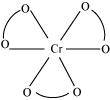(ii) [Co(NH3)3Cl3]

Two geometrical isomers are possible.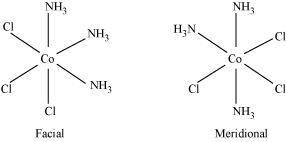#### Question 9.10:

Draw the structures of optical isomers of:

(i) [Cr(C2O4)3]3−

(ii) [PtCl2(en)2]2+

(iii) [Cr(NH3)2Cl2(en)]+

(i) [Cr(C2O4)3]3−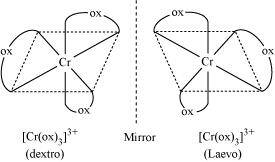(ii) [PtCl2(en)2]2+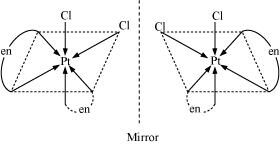(iii) [Cr(NH3)2Cl2(en)]+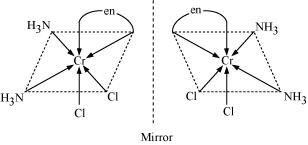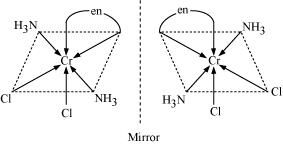Question 9.11:

Draw all the isomers (geometrical and optical) of:

(i) [CoCl2(en)2]+

(ii) [Co(NH3)Cl(en)2]2+

(iii) [Co(NH3)2Cl2(en)]+

(i) [CoCl2(en)2]+
Geometrical isomerismOptical isomerism
Since only
cis isomer is optically active, it shows optical isomerism.In total, three isomers are possible.

(ii) [Co(NH3)Cl(en)2]2+

Geometrical isomerismOptical isomerism
Since only cis isomer is optically active, it shows optical isomerism.(iii) [Co(NH3)2Cl2(en)]+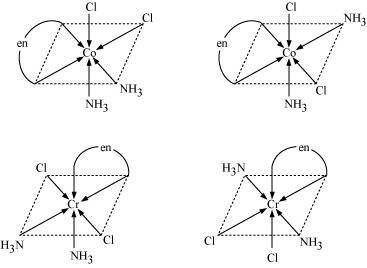#### Question 9.12:

Write all the geometrical isomers of [Pt(NH3)(Br)(Cl)(py)] and how many of these will exhibit optical isomers?

[Pt(NH3)(Br)(Cl)(py)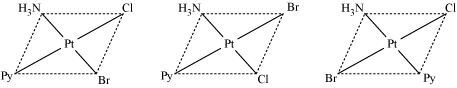From the above isomers, none will exhibit optical isomers. Tetrahedral complexes rarely show optical isomerization. They do so only in the presence of unsymmetrical chelating agents.

#### Question 9.13:

Aqueous copper sulphate solution (blue in colour) gives:

(i) a green precipitate with aqueous potassium fluoride, and

(ii) a bright green solution with aqueous potassium chloride

Explain these experimental results.